# Kerala Syllabus 9th Standard Physics Solutions Chapter 1 Forces in Fluids

You can Download Forces in Fluids Questions and Answers, Summary, Activity, Notes, Kerala Syllabus 9th Standard Physics Solutions Part 1 Chapter 1 help you to revise complete Syllabus and score more marks in your examinations.

## Kerala State Syllabus 9th Standard Physics Solutions Chapter 1 Forces in Fluids

### Forces in Fluids Textual Questions and Answers

Activity

Question 1.
Take water in a bucket. Place a tightly closed bottle on it. What do you observe?
Bottle floats on water surface

Question 2.
Immerse the plastic bottle to the bottom of the bucket. Don’t you have to exert a force? Why is it so?
It is because water exerts an upward force

Question 3.
Leave the bottle free. What do you observe?
Bottle rises to the surface of waterQuestion 4.
When an object in water is lifted, what happens? What can be the reason?
When an object in water is lifted, its weight appears diminished, when compared to that in air.
It is because water exerts an upward force on a body placed in it.
Fluids: Liquids and gases together are generally called fluids.
Buoyancy: When a body is immersed completely or partially in a liquid, the liquid exerts an upward force on the body. This force is the buoyancy.

Question 5.
Tabulate some situations in your daily life where buoyant forces are experienced in liquids and gases.

• A hydrogen-filled balloon rises in the air.
• Ships float on the surface of water.
• Submarines work by controlling the upthrust of water.
• While drawing water from well, weightlessness is felt when the bucket is underwater level.
• Life jacket floats on the surface of water.

To Measure Buoyancy?

Experiment:
Aim: To find method to measure the buoyancy experienced by a body in a liquid.
Materials: Stone, piece of metal, water, beaker, spring balance.
Procedure: Take a piece of stone and a piece of metal of almost the same size. Find out the weight of each in air using a spring balance calibrated in newton. Then find the weight of each of them when immersed in water. Record the data obtained in table.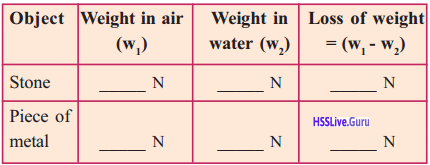Observation: The stone and the metal piece lose weight in water.
Conclusion: Buoyancy is the same as the loss of weight experience in water. That is in order to calculate the buoyancy experienced by a substance immersed in a fluid, it is enough to find out the loss of weight of the substance in that fluid.
Buopyant force of liquid = loss of weight

Factors That Influence Buoyancy

Experiment: To find the factors that influence buoyancy
Materials: Water, Kerosene, Saline water, beakers, spring balance, stone, copper block and iron block of same weight etc.
Activity 1: Take water, kerosene and saline water in three separate beakers. Find out the buoyancy of these liquids which is exerted on a piece of stone and tabulate.• The stone experiences maximum buoyancy in saline water.
• The stone experiences least buoyancy in kerosene. Density of Kerosene is lower than that of other liquids.

Analysis:

 Liquid Density (kg/m3) Water 1000 Kerosene 810 Saline water 1025 (approx.)

Saline water has greatest density and kerosene has least density. As density increases, buoyancy also increases.
Conclusion
Density of a liquid is a factor that influences the buoyancy of a body in that liquid. As density of the liquid increases buoyancy increases.
Activity: Calculate the buoyancy exerted by water on two blocks of equal weight, one of copper and the other of iron.

 Object Weight in air Weight in water Buoyancy Copper block Iron block

Observation:

• Buoyancy experienced by each block can be seen different.
• Buoyancy exerted by water on copper block is greater and on iron block is less.

Analysis: The mass and weight of the copper and the iron blocks are the same. But their volumes are different. Copper block has greater volume, and the buoyancy experienced by copper block is greater. Iron block has less volume and the buoyancy experienced by iron block is less.
Conclusion: The buoyancy acting on a body depends also on its volume.

Archimedes principle

Experiment:
Aim: To find whether there is any relation between the weight of the fluid displaced and its buoyancy when an object is in a fluid.
Materials: Store, water, spring balance, overflowing jar, beaker, iron blockActivity: Find out the buoyancy of a piece of stone and an iron block in water. Simultaneously, using an overflowing jar, find out the volume of water they dis¬placed. Find out the weight of water overflowed using a spring balance. Tabulate the results in table.
Observation:

• The loss of weight of the stone in water (buoyancy) is the same as the weight of water overflowed.
• The loss of weight of the iron block in water (buoyancy) is the same as the weight of water overflowed.

Conclusion: The buoyancy experienced by an object in a liquid will be equal to the weight of the liquid displaced by it.
Archimedes principle:
When an object is immersed partially or completely in a liquid, the buoyancy experienced by it will be equal to the weight of the liquid displaced by it.

Archimedes:
Archimedes was born in 273 BC at Syracuse, a port city in Italy. He lived during the rule of Hiero II. King Heiro commissioned a goldsmith to fabricate a crown. He commanded Archimedes to find out whether there was any impurity in the crown. This problem confounded him. He knew that the density of pure gold could be calculated by dividing the mass of the gold bar by its volume.

His confusion was how to calculate the volume without damaging the crown. When he stepped into his bath, he could see water overflowing. This made him realise that he could calculate the volume of an object, by finding the volume of the water displaced by the same. By finding the density and volume of the gold he could prove that the crown contained impurities. He was stabbed to death by a Roman soldier in 212 BC during the Punic War. This happened when he was engaged in the complicated mathematical activities related to circle.

Floatation

Activity: Make a small vessel using aluminium foil of length and breadth 10 cm each. Place this small vessel on the surface of water in a trough. Then unfold the vessel of aluminium foil. Fold it till it becomes a small piece. Then put it in water.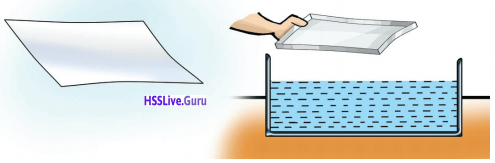Observation:

• The aluminium foil vessel floats on water.
• The folded foil sink in water.

Activity 2: Put some pieces of stone, wood, rubber cork etc., one by one into water. Note down the items which float on water. Find out the buoyancy and the weight of the water displaced by each floating body and record them in table. Observation: In the case of the floating object the buoyancy and weight of the displaced water is same.
Conclusion: A body float on a liquid when the weight of body is equal to the weight of displaced water.
Law of floatation:
Weight of a floating body is equal to the weight of the liquid displaced by it.Question 6.
Find out the reasons for the following.
a) A piece of stone experience a loss of weight within water.
b) Though an egg sinks in pure water, it floats on salt water.
c) Kerosene floats on water
d) A ship floats on water
e) When a body was placed in a liquid it remained in the same position.
a) It is because of the buoyancy exerted by water.
b) As pure water has lower density it has less buoyancy. Saline water has greater density and has greater density and has greater buoyancy. So an egg sinker in pure water and floats on saltwater.
c) Kerosene has density less than that of water.
d) It is because weight of the ship is equal to the weight of the water displaced by it.
e) Density of the body is same as that of the liquid.

Question 7.
Does a ship that enters a freshwater lake from the ocean sink more or rise move? Justify your answer.
The ship sinks more.
Seawater has more density and has higher buoyancy. Pure water has less density and less buoyancy. So the ship that enters a freshwater lake from the ocean sinks more.

Question 8.
What are the factors affecting buoyancy?
Density of the liquid and volume of the body

Question 9.
As density of a liquid increases buoyancy (increases/decrease)
increases

Relative Density

Relative density of a substance is the ratio of the density of the substance to the density of water.
Relative density = $$\frac{\text { Density of substance }}{\text { Density of water }}$$
Since it is a ratio, relative density has no unit.

Question 10.
Density of water is =
1000 kg/m3

Question 11.
How is relative density of kerosene calculated?
Relative density of a liquid = $$=\frac{\text { Density of liquid }}{\text { Density of water }}$$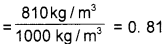Question 12.
Write examples for liquids of density greater and lesser than that of water.

 Density greater than that of water Density lesser than that of water 1. Honey 1. Kerosene 2. Saline water 2. Petrol 3. Mercury 3. Diesel

Question 13.
Which device is used to measure the relative density of a liquid?
Hydrometer

Question 14.
What will be the reading when the hydrometer is dipped in water?
1

Question 15.
Suppose the hydrometer is dipped in a liquid of density greater than that of water. Will the liquid surface be above or below the marking of 1?
The liquid surface will be below the marking of 1.

Question 16.
In which case does the hydrometer sink more? In liquids of greater density or those with a lower density?
In liquids of lower density.

Question 17.
Why do the markings on the hydrometer increase towards the bottom?
When a hydrometer is dipped in a liquid of greater density, the liquid surface will be below the marking of 1. But in a liquid of lower density, the hydrometer sinks more and the liquid surface will be above the marking of 1. Relative density of water is 1, and that of liquids with higher density will be greater than 1 and that of liquids with lower density will be less than 1.

Question 18.
Name two instruments which work on the principle of floatation?
Hydrometer:Lactometer:Question 19.
Which instrument is used to test the purity of milk?
Lactometer

Pascal’S Law

Activity 1: Take a plastic bag without holes and fill it with water. Tie its mouth tightly so that no air gets inside. Make holes on the bag with a pin. Observe what happens?
Observation: There are identical streams of water from all the holes.
Conclusion: Pressure applied at any point of the bag is transmitted equally to entire bag.
Pascal’s Law:
The pressure applied at any point of a liquid at rest in a closed system will be experienced equally at all parts of the liquid.
Volume of a liquid can not be changed using pressure. This is the basis of Pascal’s law.
Activity 2: Fill two identical syringes with water. Connect them with a plastic tube. Then arrange them as shown in figure.Question 20.
Press slightly on the end X. What do you observe.
Y rises upQuestion 21.
Bring the ends X and to the same level. Place a slotted weight of 1N (100 gwt) at the end of X. What happens at the end Y?
Y rises

Question 22.
Place a weight of 1 N at the end Y as well. What do you observe now?
X and Y balance
Then replace the syringe X with one of slightly smaller diameter and repeat the activity.

Question 23.
Are you able to balance with 1N at Y?
No
Now increase the weight at Y and find out the weight required to bring both the ends to the same level.

Question 24.
More weight was required at to attain equilibrium. Is it not due to the increased surface area of water at that end?
YesIt is the figure of a hydraulic jack. Two cylinders of different area of cross-section, filled with water, are connected using a pipe.
A1 is the area of cross-section of the smaller piston and A2, that of the bigger one.
When a force F1 is applied on the piston X, a force F2 will be experienced on the piston Y. Then according to Pascal’s Law, pressure applied on the side X will be equal to the pressure experienced on the side Y.
So Px = Py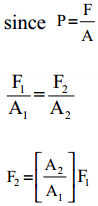If the area of cross-section of the 100 times that of the first piston
A2 = 100A,
Therefore A2/A1 = 100
F2 = 100F1
That means the large piston will experience a force of 100N when a force of 1N is applied on the small piston. The amount of work done on the two dies of the tube is equal. Therefore if the section Y is to be raised by 1cm, the section X has to be lowered by 100cm.Question 25.
Devices constructed on the basis of Pascal’s Law

• Hydraulic brake of vehicles
• Hydraulic jack
• Hydraulic press
• Excavator, Hydraulic lift

Capillarity

Activity: Take two boiling tubes, one containing water, and the other mercury. Dip a capillary tube in each tumbler.Question 26.
What happened to the water level in the capillary tube?
Water rises up in the tube
The rising of water in a tube against gravitation or against the weight of water is known as capillary rise.

Question 27.
Does the capillary rise occur in mercury as well? What did you observe?
No. depression occurs in the case of mercury.

Capillarity:
The rise or depression of a liquid in a narrow tube or a minute hole is capillarity.
Cohesive Force:
Cohesive force is the force of attraction between molecules of the same type.
Adhesive force is the force of attraction between molecules of different types of substance.

Question 28.
What is the reason for capillary rise?
Capillary rise occurs when the adhesive force is greater than the cohesive force.

Question 29.
What is the reason for capillary depression?
Capillary depression occurs when the cohesive force is greater than the adhesive force.

Question 30.
Arrange capillary tubes of different diameter on a piece of thermocol. Dip the capillary tubes in water. Compare the capillary rises in the tubes.a) Which has greater capillary rise?
In the tube of smaller / greater diameter
b) Which has lower capillary rise?
c) How is the diameter of the tube and capillary rise related?
a) In the tube of smaller
b) Larger diameter
c) As the diameter of the tube decreases, capillary rise increases. As diameter increases, capillary rise decreases.

Question 31.
Why does the capillary rise increases when the diameter of the tube decreases?
Weight of the liquid in the tube is supported by adhesive force. When the diameter of the tube decreases, the weight of the liquid it can contain also decreases. The adhesive force with the tube is greater than the cohesive force of the liquid. So the liquid is able to rise. Capillary rise increases with the decrease in the diameter.

Question 32.
Why does the capillary rise decreases when the diameter is increased?
In bigger tube, as the liquid level rises, the volume increases and the weight of the liquid also increases. Capillary rise is opposed by the weight of the liquid in the tube.Question 33.
How does the oil rise up along the wick made of cotton cloth in lamps?
This is due to capillarity. Oil rises up through the narrow capillary tubes in the cotton wick.Question 34.
Why is the land ploughed before the beginning of summer? Does it have any relation to the capillary rise?
When the land is ploughed the separation between the soil particles increases. The diameter of the capillary tubes formed in the soil increases. So the capillary rise decreases. This helps to retain moisture in the soil.

Viscous Force

Place one drop each of kerosene, water, glycerine and honey at various places along a single line at one end of a glass plate. Hold the glass plate slightly tilted. Compare the speed of flow of each liquid and writedown.

• Water flows faster than honey
• Kerosene flows faster than water
• Water flows faster then glycerine.

Conclusion: Different liquids flow at different speed. There is frictional force between the layers of each. liquid. This frictional force is parallel to the layers which try to prevent the relative motion between the layers. This frictional force is viscous force. Every layer of liquid prevents the flow of the layer is contact with it. This is the reason for the variation in speed between the layer of liquid.

Question 35.
What is viscosity?
Viscosity is the characteristic property of liquid to initiate a force that tries to prevent the relative motion between the layers of the liquid.

Question 36.
Find out liquids of viscosity greater and lower than that of water and tabulate them.Greater viscosity Lower viscosity 1. Honey 1. Kerosene 2. Glycerine 2. Diesel 3. Tar 3. Petrol 4. Mercury

Viscous liquids:
Liquids of greater viscosity are the viscous liquids, eg. honey, glycerine
Mobile liquids:
Liquids of very low viscosity are the mobile liquids eg. kerosene, diesel, petrol

Question 37.
What is the relation between temperature of a liquid and its viscosity?
As temperature of a liquid increases, its viscosity decreases

Question 38.
Why is it said that the body of a person who gets an electric shock should be massaged well?
The body temperature of a person who gets an electric shock falls suddenly. As a result, the viscosity of the blood increases, causing hindrance to the flow of blood, resulting in a heart attack. When massaged, the body becomes warm and the viscosity of the blood attains normal level. The person thus overcomes the dangerous situation.

Let Us Assess

Question 1.
The weight of a piece of stone in air is 120 N and its weight in water is 100 N. Calculate the buoyancy, experienced by the stone.
Buoyancy = weight of stone in air – weight in water = 120 N – 100 N = 20 N

Question 2.
A body which floated in water sank when put in kerosene. Why did it happen?
Kerosene has lower density than water. So kerosene can not exert more buoyancy when compared with water.

Question 3.
Observe the figures of an object placed in different liquids.a) Compare the gravitational force and the buoyancy acting on the body when it is in the liquids A and B.
b) Among A and B, which is the liquid whose density is greater than that of the object? Why?
a) In the liquid A, buoyancy is greater than the gravitational force. In B, buoyancy is less than the gravitational force,
b) Liquid A. As the body floats, the density of the liquid is greater.

Question 4.
A body of weight 1000 N sinks in water. The weight of the liquid overflowed is 250 N.
a) What will be the weight of the body in water?
b) A body of the same weight as above floats in water. What is its weight in water? What will be the weight of the water displaced?
a) Weight of the body in water = 1000 N – 250 N = 750 N
b) Zero, 1000N

Question 5.
The area of one end of a U-tube is 0.01 m2 and that of the other end the force 1 m2, When a force was applied on the liquid at the first end?
A1 = 0.01 m2
A2 = 1 m2
F1 = ?
F2 = 20000 NQuestion 6.
Write down the reason for the following:
a) Ink can be blotted with chalk.
b) Sweat can be blotted with tissue paper.
a) Due to capillarity
b) Due to capillarity

Question 7.
Which is the correct figure? Why?Fig. A
Reason: The centre portion of the liquid surface is seen higher than the edge portion. This is due to the reason that in this liquids, the cohesive force greater than the adhesive force. Insides liquids undergo capillary depression instead of capillary rise.

Question 8.
A hydraulic lift has been constructed to lift vehicles of maximum 3000 Kg. weight. The area of cross-section of the piston’s platform on which the vehicles are lifted is 580 Sq.cm. Calculate the maximum pressure experienced on the small piston.
Area = 580cm2 = 0.058m2
Force = 3000 × 10 = 30000N
Pressure = $$\frac { 3000 }{ 0.058 }$$ = 517241.36Pa

### Forces in Fluids More Questions and Answers

Question 1.
What is meant by fluids?
Liquids and gases together are called fluids

Question 2.
What are the force experienced by an object inside a fluid?
a) Weight of the object (gravitational force)
b) Buoyancy applied by the fluid

Question 3.
What is buoyancy? How is buoyancy measured?
When a body is immersed completely or partially in a liquid, the liquid exerts an upward force on the body. This force is called buoyancy.
Buoyancy will be equal to the loss of weight experienced by the body inside the liquid.
Buoyancy = Weight of the body in air – weight of the body in the liquid.Question 4.
What are the factors affecting buoyancy?

• Density of liquid
• Volume of the object

Question 5.
State Achiemes principle.
When an object is immersed partially or completely in a liquid, the buoyancy experienced by it will be equal to the weight of the liquid displaced by it.

Question 6.
The given below datas are tabulated by Gokul while conducting an experiment to prove Archimedes principles. Fill the missing parts.

 Object Weight in air (N) Weight in water (N) Loss in weight Buoyancy (N) Weight of water displaced Iron …(a)… 420 …(b)… 30 Stone 100 …(c)… 20 …(d)…

 Object Weight in air (N) Weight in water (N) Loss in weight Buoyancy (N) Weight of water displaced Iron 450 N 420 30 N 30 Stone 100 80 N 20 20 N

Question 7.
Kerosene and saline water are taken in two beakers. The same stone is immersed in both liquids.
a) In which liquid, more weight difference is experienced?
b) In which liquid, the stone experiences more buoy¬ancy?
c) Write whether the buoyancy of that liquid is more or less than that of the other liquid?
d) What is the relation between the density of a liquid and buoyancy?
a) In saline water
b) In saline water
c) More
d) Buoyancy increases with increase in density of a liquid

Question 8.
X represents kerosene and Y represents water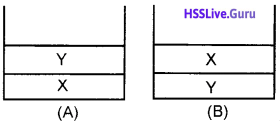Among A and B, which is the right figure? Why?
B is the right figure.
Reason: Density of water is greater than that of kerosene. When taken in the same container due to the difference in density, water comes to the lower part and kerosene to the upper part.

Question 9.
A body of weight 10 N sinks in water. Weight of the water displaced is 2N.
a) What is the weight of the object in water?
b) Which principle is related to this?
a) 8 N
b) Archimedes principle

Question 10.
State principle of floatation
Weight of a floating body is equal to the weight of the liquid displaced by it.

Question 11.
Does a ship enters a freshwater lake from a sea sink more or rise more? Justify your answer.
Sinks more
Seawater has more density. Seawater exerts more buoyancy on the ship. Freshwater has less density. It exerts less buoyancy.

Question 12.
An object of weight 500 N sinks in water. Weight of water overflowed is 50 N.
a) What is the weight of the object in water?
b) If another object of the same weight floats on water, what is the weight in water? What is the buoyancy? What is the loss of weight in water?
a) Weight in water = Weight in air – weight of water overflowed = 500 N – 50 N = 450 N
b) When floats,
Weight in water = 0 N
buoyancy = 500 N
Loss of weight in water = 500 N
(When a body floats on a liquid, weight of the body in air = loss of weight = buoyancy = weight of water displaced)

Question 13.
Density of water is = ………….
1000 kg/m3

Question 14.
Relative density of water = ………
1

Question 15.
Classify the following liquids into liquids having density greater than that of water and liquids having density less than that of water.
Honey, kerosene, glycerine, mercury, saline water, petrol, diesel

 Liquids having density greater than that of water Liquids having density less than that of water 1. Honey 1. Kerosen 2. Glycerine 2. Petrol 3. Mercury 3. diesel 4. Saline water

Question 16.
What is the relation between the diameter of capillary tube and capillary rise? Why?
As the diameter of the tube increases, capillary rise decreases.
Weight of the liquid inside the tube opposes capillary rise.

Question 17.
On which law, hydraulic break works? Name other three devices that works on the basis of this law. State the law.
Hydraulic break works on the basis of Pascal’s law. Other devices are hydraulic jack, hydraulic press and excavator.
Pascal law: The pressure applied at any point of a liquid at rest in a closed system, will be experienced equally at all parts of the liquid.

Question 18.
Honey : Viscous liquid
Petrol : ……………
Mobile liquidQuestion 19.
P, Q and R are three capillary tubes. If these are dipped in water.a) Which has greater capillary rise?
b) Which factor influenced capillary rise?
c) Why is the land ploughed before the beginning of summer?
a) In R
b) Diameter of the tube
c) When the land is ploughed the separation between the soil particles increases. The diameter of the capillary tubes formed in the soil increases. So the capillary rise decreases. This help to retain moisture in the soil.

Question 20.
In the given figure, the area of cross-section of B is 10 times greater than that of A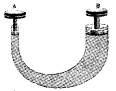a) What is the working principle behind this device?
b) If a force of 20 N is applied on A, what will be the force experienced on B?
c) If the section A is lowered by 1 cm, how much will B rise up?
a) Pascal’s lawc) Rises 10 cm

Question 21.
An iron block of weight 2N is immersed in three dif-ferent liquids A, B, C weight of the iron block experi-enced inside the liquids are labelled in the figure.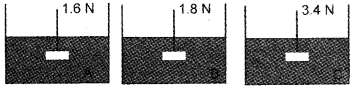a) In which liquid, the iron block experiences more buoyancy?
b) Among these three liquids, which has less density?
c) What is the relation between density of a liquid and buoyancy?
a) C
b) B
c) As density of the liquid increases, buoyancy also increases.

Question 22.
When a hydrometer is dipped n a liquid, the reading is found to be 1.25. What is the density of the liquid?
Relative density = $$\frac{\text { density of liquid }}{\text { density of water }}$$
∴ Density of liquid = Relative density x density of water
= 1.25 × 1000 = 1250 kg/m3

Question 23.
What is the relation between viscosity and temperature?
Viscosity decreases with increase in temperature.

Question 24.
Whan a midrib of a coconut leaf, pencil etc. are dipped in water, and taken out, water is seen sticking to them. Why?
Due to the adhesive force between the midrib of a coconut leaf and water.

Question 25.
When a piece of chalk is used on a blackboard, particles of the chalk stick to the board. Why?
Due to the adhesive force between particles of chalk and particles of board.Question 26.
Fingers are wetted at intervals when currency notes are being counted. Why?